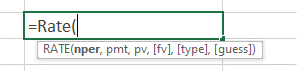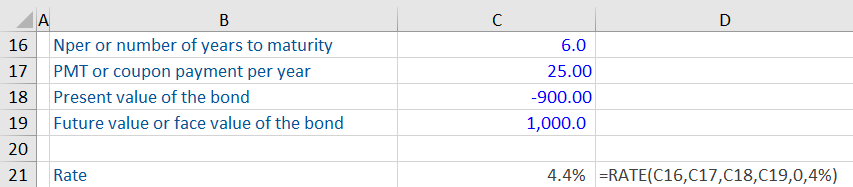## What is the “RATE Function”?

The RATE function is a financial function in Excel that calculates the interest rate per period of an annuity. The function is used to calculate the periodic interest rate, which can then be multiplied as required to calculate the annual interest rate. The function calculates by iteration.

The function can be used to calculate the interest rate charged by a loan or offered by an investment for a given period. The RATE function’s output can help decide whether to borrow at a particular interest rate or invest in a security, such as bonds, at the rate of return offered.

## Key Learning Points

• The RATE function is a financial function in Excel used for calculating the interest rate of a loan or an investment for a given period. The function can be applied to loans to calculate the percentage of interest charged or to investments to calculate the percentage of return offered
• While interest rate can also be calculated without the RATE function, it makes the calculation more efficient
• The RATE function gives its output through trial and error. If the results do not converge within 20 iterations, it presents a #NUM! error in Excel
• The function returns a #Value! error if any of the supplied arguments are non-numeric

## Syntax

The syntax for the RATE function is shown as:

Rate(nper, pmt, pv, [fv], [type], [guess]The syntax includes the following arguments:

• Nper represents the number of payment periods for which the interest rate is being calculated. The periods can be in months, quarters, or years. The output of the RATE function (the interest rate) is based on the period included in this argument. The annualized return can be calculated from the period return.
• Pmt is the amount of payment in each period. This number must be constant over the loan’s/investment’s life. If Pmt is omitted, the FV value should be entered.
• PV is the present value of the future payments. For example, if it is a loan, the PV is the amount borrowed. If it is an investment, the PV is the amount invested today.

### Optional Arguments

• FV is the future value or the cash balance after the last payment is made. If it is a loan, the FV will be 0. If it is an investment, the FV will be the desired amount of cash flow resulting from the payments. If FV is omitted, it is assumed to be 0.
• Type indicates the payment due dates. If the payment is to be made at the end of each period, the type is 0. If payment is due at the beginning, the type is 1.
• Guess is a guess of what the interest rate is likely to be. If omitted, the rate is assumed to be 10%.

## Important Points about the RATE Function

• The RATE function calculates the rate with an iterative process (trial and error). It will return a #NUM! error value if the results do not converge within 20 iterations. The guess provides a starting point for the rate function to converge an answer sooner before reaching 20 iterations. If RATE does not converge, try and input other values in the guess argument.
• #NUM! error value may also be returned due to errors related to cash flow conventions. Typically, cash outflows are input with a negative sign.
• #VALUE! error happens if any of the arguments are non-numeric.
• The Nper argument should be consistent with the payment. For example, if it is a 5-year loan with an interest payment of 200 per month, the NPER should 60 (5 years x 12 months). Also, the units used for Nper and guess should be consistent.
• The output given by the RATE function depends on the period input for loan payments. For example, if the NPER is 12 and the input in PMT is monthly payments, the RATE function’s output will be a monthly interest rate. If you want the annual interest rate, you need to multiply the monthly rate by 12.

## Example 1:

Based on the information below, we have been asked to calculate the interest rate for this loan using Excel’s RATE function. Download this free rate function workout from our blog and practice yourself.The RATE function has calculated the interest rate for this loan as 0.6%. The payment per month is entered as a negative number to reflect a cash outflow. This output is the monthly interest rate as the Nper and payments are monthly. If you want the annual interest rate, you need to multiply this output by 12.

## Example 2:

Calculate the yield to maturity or the return rate offered by the bond below. Coupon payments are made at the end of each year.The bond gives an annualized return of 4.4%. Notice we have also included the optional arguments, including a guess of the interest rate as 4%. The present value is a negative number as investing in the bond today will lead to a cash outflow. Investing in a bond is different from taking a loan, where first there is a cash inflow, followed by outflows towards interest and principal repayment. In a bond, in the beginning, there is a cash outflow (the investment), followed by cash inflows (interest payments and eventually the original investment).